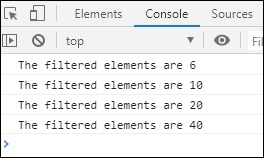# RxJS - Filtering Operator filter

This operator will filter the values from source Observable based on the predicate function given.

## Syntax

```filter(predicate_func: function): Observable
```

## Parameters

predicate_func − The predicate_func, will return a boolean value, and the output will get filtered if the function returns a truthy value.

## Return value

It will return an observable with values that satisfies the predicate_func.

## Example

```import { of } from 'rxjs';
import { filter } from 'rxjs/operators';

let all_nums = of(1, 6, 5, 10, 9, 20, 40);
let final_val = all_nums.pipe(filter(a => a % 2 === 0));
final_val.subscribe(x => console.log("The filtered elements are "+x));
```

We have filtered the even numbers using filter() operator.

## Output